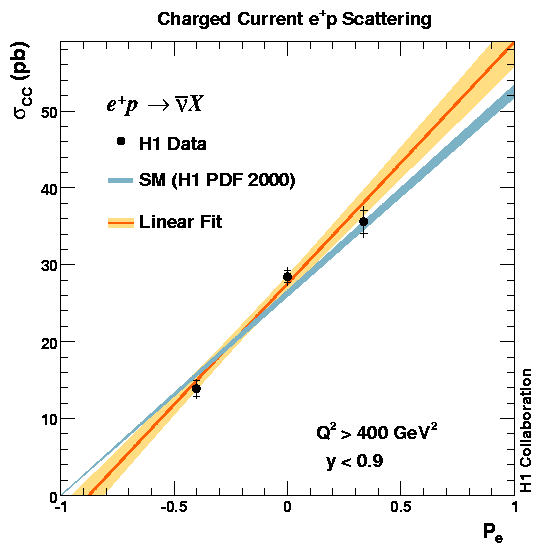# First Measurement of Charged Current Cross Sections at HERA with Longitudinally Polarised Positrons

In an electron (or a positron) storage ring like HERA, transverse polarisation of the e-/+ beam arises naturally through synchrotron radiation via the Sokolov-Ternov effect. Longitudinal polarisation at the H1 e-/+p interaction point is available since 2003. It is obtained by using a set of spin rotator magnets; these are basically vertically-deflecting magnets whose length is set to give a spin precession of 90o into the longitudinal direction, and then reverse it back to vertical after the interaction region. The value of longitudinal polarisation is defined by Pe = (NR - NL)/(NR + NL) with NR (NL) being the number of right (left) handed e-/+ in the beam.

The charged current (CC) process studied here proceeds via weak interactions with an exchange of a W boson in t channel. Unlike a photon or a Zo boson exchanged in neutral current interactions, the W boson interacts only with e-L (or e+R) in the Standard Model (SM). Therefore the SM CC cross section is expected to have a linear dependence on Pe and vanishes for a fully right (left) handed electron (positron) beam.In this Letter first measurements of the charged current total cross sections e+p ® nbar X are reported for two values of longitudinal polarisation Pe of the positrons. These measurements use data collected in 2003-2004. This is the first publication based on the HERA II data. The corresponding data sets are termed the R and L data sets respectively. The R data set has a luminosity weighted mean polarisation value of (33.6+/-0.7)% and an integrated luminosity value of 26.9+/-0.6 pb-1. The corresponding numbers for the L data set are (-40.2+/-1.1)% and 20.7+/-0.5 pb-1. In both data sets the incident positron beam energy is 27.5GeV, whilst the unpolarised proton beam energy is 920GeV. This yields a centre-of-mass energy of 318GeV.

These measurements, as well as the corresponding one obtained using the published unpolarised 65.2 pb-1 data collected in 1999 and 2000, are compared (figure) to SM expectations based on the H1 PDF 2000 parametrisation. A linear fit to the polarisation dependence of the measured cross sections is performed taking into account the correlated systematic uncertainties between the measurements. The fit provides a reasonable description of the data with a chi2 value of 4.4 for one degree of freedom. The result of the fit extrapolated to the point Pe = -1 yields a fully left handed charged current cross section of -3.9 +/- 2.3stat +/- 0.7sys +/- 0.8pol pb. The polarisation dependence of the charged current cross section has thus been established at HERA and the data are found to be in agreement with the SM axiomatic absence of right handed charged currents.

Last Update 06.02.2006